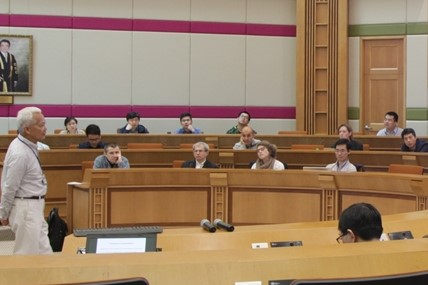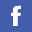## Colloquium/Seminar

 Year Month 2023 Jan     Feb     Mar     Apr     May     Jun     Jul     Aug     Oct 2022 Jan     Feb     Mar     Apr     May     Jun     Jul     Aug     Oct     Nov     Dec 2021 Feb     Mar     Apr     May     Jun     Jul     Aug     Sep     Oct     Nov     Dec 2020 Jan     May     Jun     Jul     Aug     Sep     Oct     Nov     Dec 2019 Jan     Feb     Mar     Apr     May     Jun     Jul     Aug     Oct     Nov

### Event(s) on July 2022

• Thursday, 14th July, 2022

 Title: Statistical Learning for Kernel-Based Functional Linear Regression Speaker: Ms GUO Keli , Department of Mathematics, Hong Kong Baptist University, Hong Kong Time/Place: 14:30  -  16:30 Zoom, Meeting ID: 923 0328 0124 Passcode: 517988 Abstract: Over the last two decades, functional linear regression that relates a scalar response on a functional predictor has been extensively studied. In practice, however, apart from functional predictors, scalar predictors or outliers are frequently included in the dataset. To address this issue, we investigate three variants of the functional linear regression model within the framework of reproducing kernel Hilbert space (RKHS), respectively. First, we consider the semi-functional linear model that consists of a functional component and a nonparametric component. A double-penalized least squares method is adopted to estimate both the functional and nonparametric components within the framework of reproducing kernel Hilbert space. By virtue of the representer theorem, an efficient algorithm that requires no iterations is proposed to solve the corresponding optimization problem, where the regularization parameters are selected by the generalized cross-validation criterion. Moreover, we establish minimax rates of convergence for prediction in the semi-functional linear model. Our results reveal that the functional component can be learned with the minimax optimal rate as if the nonparametric component were known. Numerical studies and real data analysis are provided to demonstrate the effectiveness of the method and to verify the theoretical findings. Then we consider the partially functional linear regression model (PFLM) that consists of a functional linear regression component and a sparse high-dimensional linear regression component. We adopt a double-penalized least squares approach to estimate the functional component within the framework of reproducing kernel Hilbert space and the parametric component by sorted l_1 penalized estimation (SLOPE). Moreover, we establish minimax rates of convergence for prediction in the PFLM. Our results suggest that the estimator obtained by SLOPE can achieve the minimax optimal rate regardless of the functional component. In contrast, the learning rate for the functional component depends on both functional and parametric components. To solve the optimization problem, an efficient computing algorithm is proposed with the help of the representer theorem. Numerical studies are conducted to demonstrate the performance of the proposed method. Finally, we propose an outlier-resistant functional linear regression model so that we can perform robust regression and outlier detection simultaneously. The proposed model includes a subject-specific mean shift parameter in the functional linear regression model to indicate whether an observation is an outlier or not. We adopt a double-penalized least squares method to estimate the functional component within the framework of reproducing kernel Hilbert space and the mean shift parameter by l_1 penalization or SLOPE. By virtue of the representer theorem, an efficient algorithm is proposed to solve the corresponding optimization problem. Moreover, we establish the minimax rates of convergence for prediction and estimation in the proposed model. Our results reveal that the convergence rate for estimation of the mean shift parameter is not affected by the functional component. The functional component can be learned with the minimax optimal rate as if there were no outliers. Numerical studies are provided to demonstrate the effectiveness of the proposed methods.

• Monday, 25th July, 2022

 Title: An Operator Splitting Method for Solving Elastica Model Speaker: Ms TAO Linlin, Department of Mathematics, Hong Kong Baptist University, Hong Kong Time/Place: 15:30  -  17:30 Zoom, Meeting ID: 970 7910 9849 Passcode: 096239 Abstract: Elastica energy has a long history in variational image processing. It is known to possess a strong capability of preserving edge information for objects as well as smoothing piece-wise regions. However, it is challenging to solve the corresponding non-differential and non-convex functional. The main purpose of this dissertation is to propose an efficient algorithm based on the operator splitting scheme to solve the elastica model. Firstly, we will give a brief introduction of the operator splitting method. We will show the motivation of the operator splitting method, and then the two most commonly used operator splitting methods will be introduced. Finally, a full-time discretization scheme Marchuk-Yanenko operator splitting scheme will be introduced. Secondly, an efficient algorithm based on the operator splitting scheme is proposed to solve the \$L^2\$ elastica minimization problem. Elastica minimization problem is converted to an initial value problem by the optimality system, and then three sub-problems derived from splitting schemes need to be solved. Their simplicity guarantees the numerical implementation can be easily carried out, resulting in closed-form solutions and fast dedicated solvers. Besides, we apply our proposed method to image inpainting and segmentation. Experiment results show the efficiency and stability of our method. Thirdly, we propose an operator splitting method to solve the \$L^1\$ elastica model. Technically, it is developed by introducing auxiliary variables, employing an operator splitting method to an initial value problem. Then a series of sub-problems with Marchuk-Yanenko operator splitting scheme can be solved easily by closed-form solutions or fast dedicated solvers. Experiments on image smoothing and segmentation display signiﬁcant gains of the proposed approach.

All years### Conferences and Workshops

We organize conferences and workshops every year. Hope we can see you in future.### Lecture Series

Details### Employment